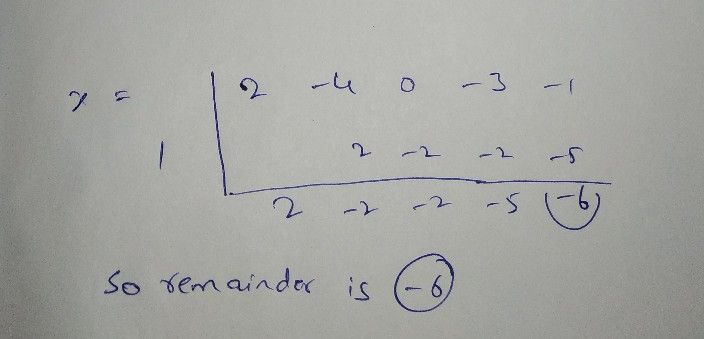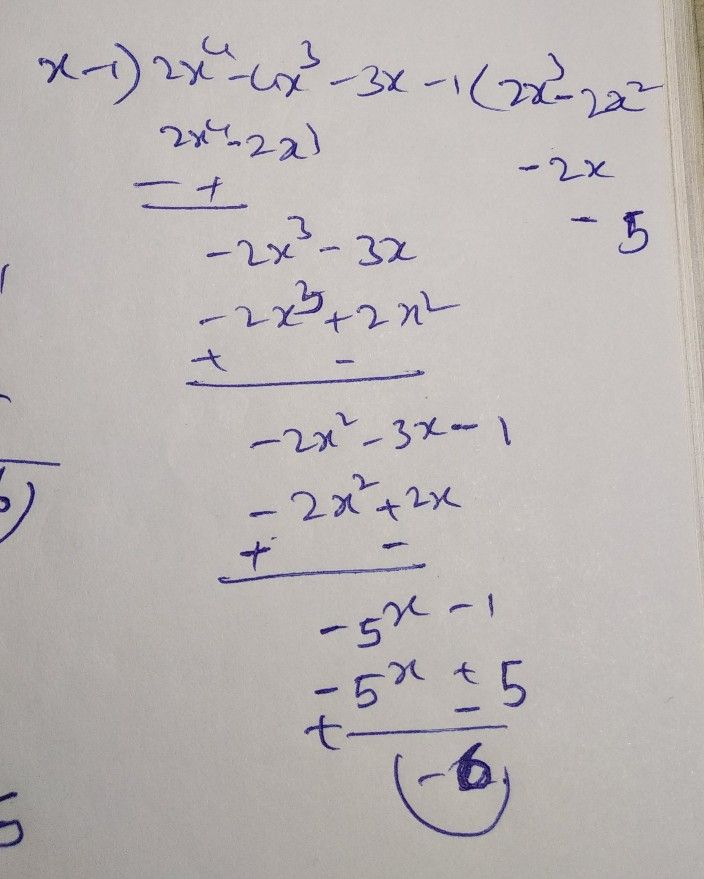Symbol
Problemthe remainder obtained on dividing $\left(x\right)-3x^{2}+x-1$ $4$ the $f\left(10$ of $x+1.10x$ $1$ t us consider some more examples. $7$ Divide the polynomial $442x^{4}-4x^{2}-3x-1$ $x$ and verify the remainder with zero of the divisor. $111$ $f\left(x\right)=2x^{4}-4x^{2}-3x-1$ st see how many times $2x^{4}$ is of x. $\dfrac {2x^{4}} {x}$ $2$ w multiply $\left(x-1\right)\left(2x^{3}\right)-2x^{4}-2x^{3}$ again sce the first term of the remainder that